Abhishek Kumar Category: Data StructuresTags: datastructures linkedlist

A Linked List or Singly Linked List is a linear data structure which collectively represents a sequence of data.
In this tutorial, we will learn about the implementation of a singly linked list and various operations on it like insertion, deletion and traversal of a Linked List.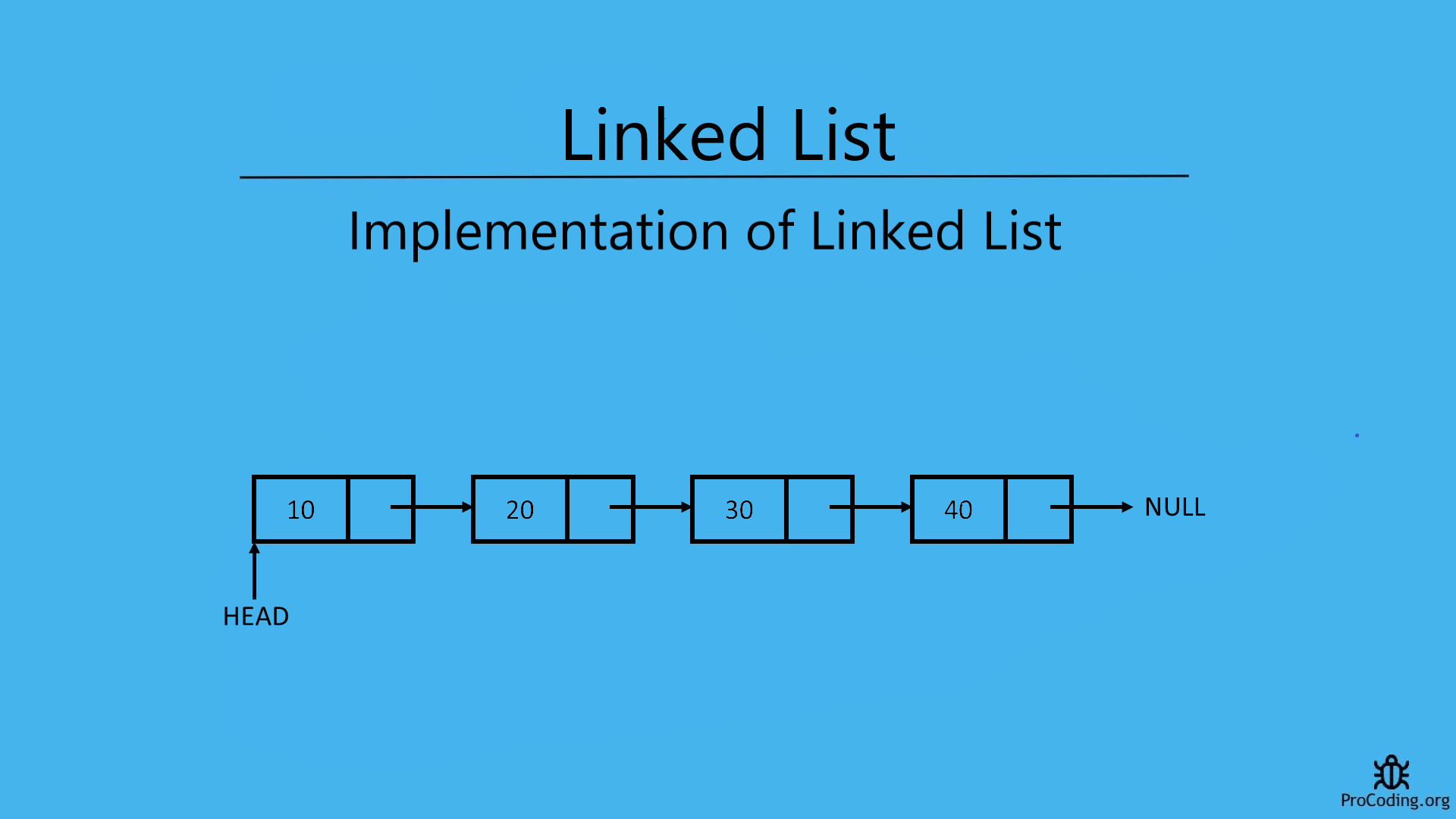A Linked List (also known as Singly Linked List) is a collection of nodes in which each node is pointing to next node and last node points to `null`.### Declaration of type node for Linked List

We have to create a data structure or Node which contains two part i.e, first part will contain the data to be stored and second will store address of next such node. We can create this by using user defined data type.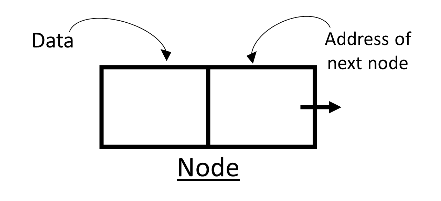``````class Node:
def __init__(self, data):
self.data = data
self.next = None``````
``````class Node {
constructor(data) {
this.data = data;
this.next = null;
}
}``````

Traversing means visiting all the node once i.e, we want to read all the data available in the linked list.
We can do so by visiting all the nodes one by one and reading data available in each node.

Simply, use a temporary node and initialize it with the head node so that we can start with the begining of the list and then go through all the nodes one by one till the last node is reached. And we can get the last node by checking the next value of node i.e., if next value of node is `null` then this is the end of node.``````def traverse(self):

while temp is not None:
print(temp.data)
temp = temp.next
else:
print(None)``````
``````traverse() {
while (temp != null) {
console.log(temp.data);
temp = temp.next;
}
} else {
console.log(null);
}
}``````

### Length of a Linked List

To find length of a linked list we can simply use a temporary variable and initialize it by assigning the head node. Now, move it to every node one by one and increase the counter variable.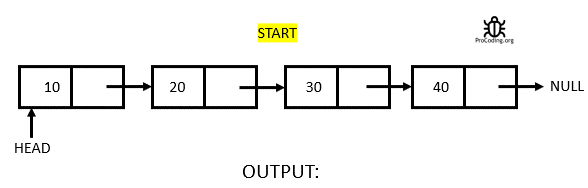``````def length(self):
count = 0
while temp:
temp = temp.next
count += 1
return count``````
``````length() {
let count = 0;
while (temp) {
temp = temp.next;
count += 1;
}
return count;
}``````

### Insertion in a Linked List

Insertion in a Linked List can be done in following three ways:

##### Insertion at the begining of the Linked List

While inserting a node at the begining position, two situation may arise:

1. Insertion when Linked List is emtpy: In this case we can simply create a new node and put the value in data part and null into the next part (which is already done in Class Node constructor) then assign it to the head.
2. Insertion when Linked List is not empty: In this case create a new node, add value into the data part and assign the node pointed pointed by head to next of newly created node and then head will now point to new node.
3.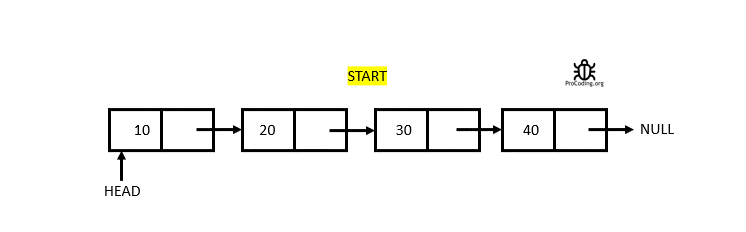``````def insert_at_start(self, data):
new_node = Node(data)
``````insertAtStart(data) {
const newNode = new Node(data);
}``````
##### Insertion at the end of the Linked List

While inserting a node at the end position, two situation may arise:

1. Insertion when Linked List is emtpy: In this case we can simply create a new node and put the value in data part and null into the next part (which is already done in Class Node constructor) then assign it to the head.
2. Insertion when Linked List is not empty: In this case create a new node, and then traverse till the end of the Linked List then add the new node in the next part of last node and put null into the next part because it is the last node now.
3.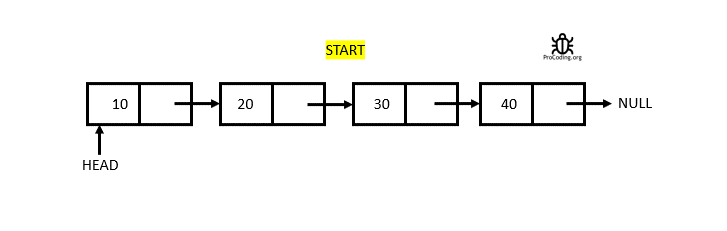``````def insert_at_end(self, data):
new_node = Node(data)
while temp.next:
temp = temp.next
temp.next = new_node
else:
``````insertAtEnd(data) {
const newNode = new Node(data);
while (temp.next) {
temp = temp.next;
}
temp.next = newNode;
} else {
}
}``````
##### Insertion at given position in the Linked List

While inserting at any given position following 3 situation may arise based on user input:

1. Insertion at the begining: In this case, simply call the function used to insert at begining.
2. Insertion at the end: In this case, simply call the function used to insert at the end.
3. Insertion at any random position: In this case, use a temporary variable and initialize with the head pointer traverse till the `given position - 1` and then insert the new node by adjusting the next pointers.
4.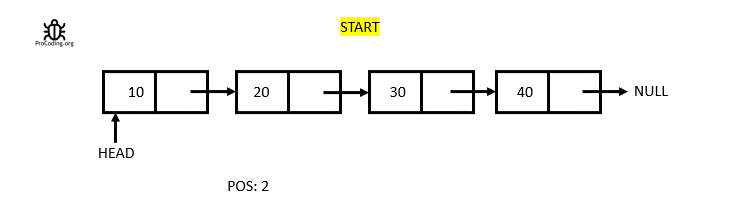``````def insert_at_position(self, pos, data):
if pos < 0 or pos >= self.length():
raise 'Enter a valid postion'
else:
if pos == 0:
self.insert_at_start(data)
elif pos == self.length()-1:
self.insert_at_end(data)
else:
for _ in range(pos-1):
temp = temp.next
new_node = Node(data)
new_node.next = temp.next
temp.next = new_node``````
``````insertAtPosition(pos, data) {
if (pos < 0 || pos >= this.length()) {
throw "Enter a valid position";
} else {
if (pos === 0) {
this.insertAtStart(data);
} else if (pos === this.length() - 1) {
this.insertAtEnd(data);
} else {
for (let i = 0; i < pos; i++) {
temp = temp.next;
}
let newNode = new Node(data);
newNode.next = temp.next;
temp.next = newNode;
}
}
}``````

### Deletion in a Linked List

Same as insertion deletion in a Linked List can be done in 3 ways:

##### Deletion from the begining of the Linked List

While deleting a node at the from begining, two situation may arise:

1. Deletion when Linked List is emtpy: When Linked List is empty throw an error that Linked List is empty.
2. Deletion when Linked List is not empty: When Linked List is not empty simply shift the head pointer to the next element.
For languages like Python, Java etc. which have in built mechanism for garbage collection, can remove the deleted node itself but in case of languages like C or C++ we have to point that node and remove it manually.
3.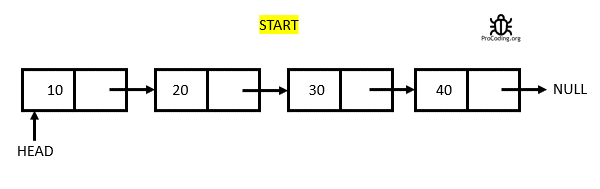``````def delete_from_start(self):
return data
else:
``````deleteFromStart() {
return data;
} else {
}
}``````
##### Deletion from the end of the Linked List

While deleting a node at the from the end, two situation may arise:

1. Deletion when Linked List is emtpy: When Linked List is empty throw an error that Linked List is empty.
2. Deletion when Linked List is not empty: When Linked List is not empty traverse the linked list till the second last element and then put `null` into the next part of this node so that its link with last element can broken.
For languages like Python, Java etc. which have in built mechanism for garbage collection, can remove the deleted node itself but in case of languages like C or C++ we have to point that node and remove it manually.
3.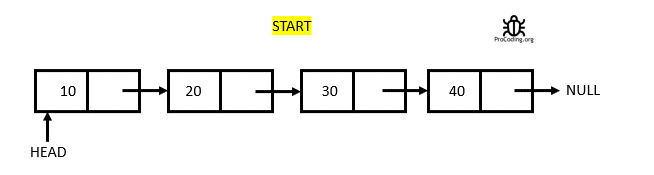``````def delete_from_end(self):
while current.next != None:
previous = current
current = current.next
previous.next = None
return current.data
else:
``````deleteFromEnd() {
while (current.next != null) {
previous = current;
current = current.next;
}
previous.next = null;
return current.data;
} else {
}
}``````
##### Deletion from given position in the Linked List

While deleting at any given position following 3 situation may arise based on user input:

1. Deletion from the begining: In this case, simply call the function used to delete from begining.
2. Deletion from the end: In this case, simply call the function used to delete from the end.
3. Deletion from any random position: In this case, use two temporary variable and initialize with the head pointer traverse one pointer till the `given position` and the other till `given position - 1` and then delete that node and then adjust the pointers.
For languages like Python, Java etc. which have in built mechanism for garbage collection, can remove the deleted node itself but in case of languages like C or C++ we have to point that node and remove it manually.
4.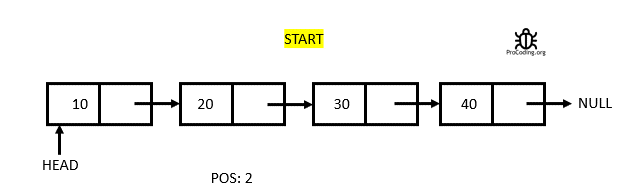``````def delete_at_position(self, pos):

if pos < 0 or pos >= self.length():
raise 'Enter a valid postion'
else:
if pos == 0:
return self.delete_from_start()
elif pos == self.length()-1:
return self.delete_from_end()
else:
for _ in range(pos):
previous = current
current = current.next
data = current.data
previous.next = current.next
return data``````
``````deleteAtPosition(pos) {

if (pos < 0 || pos >= this.length()) {
throw "Enter a valid position";
} else {
if (pos === 0) {
return this.deleteFromStart();
} else if (pos === this.length() - 1) {
return this.deleteFromEnd();
} else {
for (let i = 0; i < pos; i++) {
previous = current;
current = current.next;
}
let data = current.data;
previous.next = current.next;
return data;
}
}
}``````

Implementation of a singly linked list with all the function combined with output.

``````class Node:
def __init__(self, data):
self.data = data
self.next = None

def __init__(self):

def insert_at_start(self, data):
new_node = Node(data)

def insert_at_end(self, data):
new_node = Node(data)
while temp.next:
temp = temp.next
temp.next = new_node
else:

def insert_at_position(self, pos, data):
if pos < 0 or pos >= self.length():
raise 'Enter a valid postion'
else:
if pos == 0:
self.insert_at_start(data)
elif pos == self.length()-1:
self.insert_at_end(data)
else:
for _ in range(pos-1):
temp = temp.next
new_node = Node(data)
new_node.next = temp.next
temp.next = new_node

def delete_from_start(self):
return data
else:

def delete_from_end(self):
while current.next != None:
previous = current
current = current.next
previous.next = None
return current.data
else:

def delete_at_position(self, pos):

if pos < 0 or pos >= self.length():
raise 'Enter a valid postion'
else:
if pos == 0:
return self.delete_from_start()
elif pos == self.length()-1:
return self.delete_from_end()
else:
for _ in range(pos):
previous = current
current = current.next
data = current.data
previous.next = current.next
return data

def get_first(self):
else:

def get_last(self):
while current.next != None:
current = current.next
return current.data
else:

def get_at_position(self, pos):
if pos < 0 or pos >= self.length():

for _ in range(pos):
current = current.next
return current.data

def traverse(self):

while temp is not None:
print(temp.data)
temp = temp.next
else:
print(None)

def length(self):
count = 0
while temp:
temp = temp.next
count += 1
return count

ll.insert_at_end(1)
ll.insert_at_end(2)
ll.insert_at_end(3)
ll.insert_at_end(4)
ll.insert_at_end(5)
ll.traverse()``````
``````class Node {
constructor(data) {
this.data = data;
this.next = null;
}
}

constructor() {
}

insertAtStart(data) {
const newNode = new Node(data);
}

insertAtEnd(data) {
const newNode = new Node(data);
while (temp.next) {
temp = temp.next;
}
temp.next = newNode;
} else {
}
}

insertAtPosition(pos, data) {
if (pos < 0 || pos >= this.length()) {
throw "Enter a valid position";
} else {
if (pos === 0) {
this.insertAtStart(data);
} else if (pos === this.length() - 1) {
this.insertAtEnd(data);
} else {
for (let i = 0; i < pos; i++) {
temp = temp.next;
}
let newNode = new Node(data);
newNode.next = temp.next;
temp.next = newNode;
}
}
}

deleteFromStart() {
return data;
} else {
}
}

deleteFromEnd() {
while (current.next != null) {
previous = current;
current = current.next;
}
previous.next = null;
return current.data;
} else {
}
}

deleteAtPosition(pos) {

if (pos < 0 || pos >= this.length()) {
throw "Enter a valid position";
} else {
if (pos === 0) {
return this.deleteFromStart();
} else if (pos === this.length() - 1) {
return this.deleteFromEnd();
} else {
for (let i = 0; i < pos; i++) {
previous = current;
current = current.next;
}
let data = current.data;
previous.next = current.next;
return data;
}
}
}

traverse() {
while (temp != null) {
console.log(temp.data);
temp = temp.next;
}
} else {
console.log(null);
}
}

length() {
let count = 0;
while (temp) {
temp = temp.next;
count += 1;
}
return count;
}
}

ll.insertAtEnd(1);
ll.insertAtEnd(2);
ll.insertAtEnd(3);
ll.insertAtEnd(4);
ll.insertAtEnd(5);
ll.traverse();``````

#### Output

Output

```1
2
3
4
5```

AUTHOR### Abhishek Kumar

Software engineer | Blogger | Keen learner
Python | Django | JavaScript | React | Next.js | Express.js | C
Passionate about learning and experimenting with new things and open to more opportunities and collaborations.